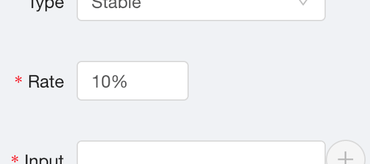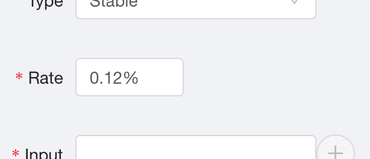# Number.prototype.toString() is incredibly useful to display numbers

04 January 2019   0 comments   JavaScript

tl;dr; Use `Number.prototype.toString()` to display percentages that might be floating point numbers.I started writing a complicated solution but as I discovered corner cases and surprised I was brutally forced to do some research and actually read some documentation. Turns out `Number.prototype.toString()`, with the `precision` argument omitted, is the ideal solution.

The application I was working on has an input field to type in a percentage. I.e. a number between 0 and 100. But whatever the user types in, we store the number in decimal. So, if the user typed in "10" into the input widget, we actually store it as `0.1` in the database. Most people will type in a whole number (aka. an integer) like "12" or "5" but some people actually need more precision so they might type in "0.2%" which means `0.002` stored in the backend database.

But the widget is a React controlled component meaning it's `value` prop needs to be potentially formatted to what gives the best user experience. If the user types in whole numbers set the `value` prop to a whole number. If the user types in floating point numbers set the `value` prop type a floating point number with the "matching formatting".I started writing an overly complicated function that tries to figure out how many decimal-points the user typed in. For example `0.123` is 3 because `parseInt(0.123 * 10 ** 3, 10) === 0.123 * 10 ** 3`. But, that approach doesn't work because of floating point arithmetic and the rounding problem. For example `103441 !== 10.3441 * (10 ** 4) === 103440.99999999999`. So, don't look for a number to pass into `.toFixed()`.

Turns out `Number.prototype.toString()` is all you need. If you omit the precision argument, it figures out how many significant digits to use based on the input. It's best explained with some examples:

```> (33).toString()
"33"
> (33.3).toString()
"33.3"
> (33.10000).toString()
"33.1"
> (10.3441).toString()
"10.3441"```

Perfect!

### Next level stuff

So actually, it's a bit more complicated than that. You see, the number stored in the backend database might be `0.007` which you and I know as "0.7%" but be warned:

```> 0.008 * 100
0.8
> 0.007 * 100
0.7000000000000001```

You know, because of floating-point arithmetic, which every high-level software engineer remembers understanding one time years ago but now know just to watch out for.

So if you use the `toString()` on that you'd get...

```> var backendPercentage = 0.007
> (100 * backendPercentage).toString() + '%'
"0.700000000000001%"```

Ouch! So how to solve that? Use `Math.round(number * 100) / 100` to get rid of those rounding errors. Apparently, it's very fast too. So, now combine this with the `toString()`:

```> var backendPercentage = 0.007
> (Math.round(100 * backendPercentage * 100) / 100).toString() + '%'
"0.7%"```

Perfect!